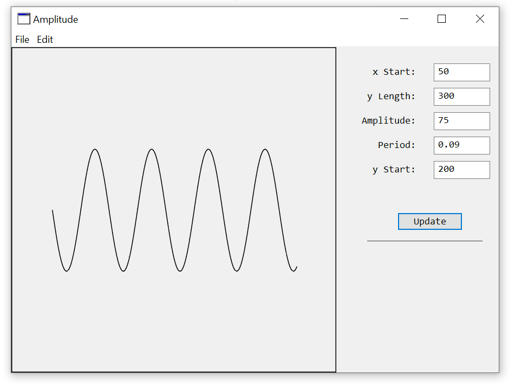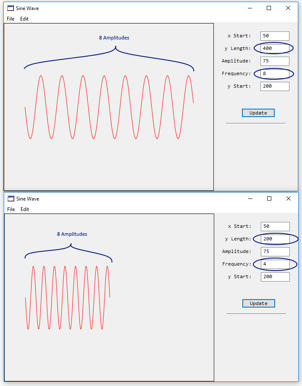# Draw wavy lines

Wavy lines are usually treated as sinus curves. I don’t know anything about this field at all.
What would I like to do? My goal is to draw a wavy line (see figure) in the Graphics object where the start and end coordinates are known. So xStart, yStart and xEnd and yEnd. I’d like to have a method DrawWavyLine(g As Graphics, x As Double, y As Double, x2 As Double, y2 As Double). I would fix the amplitude in the method (using a constant). Has any of you ever done this before? Is there a resource-saving algorithm so you don’t have to learn a sequence of CurveShapes? Thanksyou need the amplitude (which you say you have) but is actually (y2-y1)
the start and end points (which you have)
but also you need the number of “waves” or degrees per X value (each peak is a cycle [you have 3 in your example)

the rest is basic math where y is SIN(a) and A is incremented by a double value …

Its too early for me to work out all the math here… but its not rocket science… it IS a bit of simple Trig

I actually only have the width of the object and its start coordinates to create the wavy line. I want to draw the wavy line under the object. Here’s a Xojo method. But it is not optimized for my wishes. I don’t know where to insert the given width so that it is taken into account in the calculations. Furthermore, I’m not sure whether calling the DrawLine method dozens of times is a computationally sensitive variant.

[code]Const kyEx = 100 ’ spacing from the top
Const keF = 2 ’ size multiplier

Dim x1, y1, y2 As Double

g.ForeColor = TextColor

For x As Double = 0 To 40 Step 0.1 ’ here i need to a value to draw the linbe to the given width i want
y2 = Sin(x)
g.DrawLine(x1 * keF, y1 * keF + kyEx, x * keF, y2 * keF + kyEx)
x1 = x
y1 = y2
Next[/code]

While this old code needs a couple tweaks (create a picture to get a graphics onbject and in the open event just comment the line out) after that it runs just fine. The line editor does have an example for drawing wavy lines… and it seems plenty fast enough

http://katkosoft.com/StyledLine/Styledlines.html

For some other sample code, check out:

Examples/Graphics and Multimedia/GraphTest

[quote=442375:@Karen Atkocius]While this old code needs a couple tweaks (create a picture to get a graphics onbject and in the open event just comment the line out) after that it runs just fine. The line editor does have an example for drawing wavy lines… and it seems plenty fast enough

http://katkosoft.com/StyledLine/Styledlines.html[/quote]
Thanks @Karen Atkocius . I took a look on it, but its bit to complex for me at the moment.

What needs to be modify at my code above to calculate the wavy line between two given points?

Hi Martin,

Waves do get a little more complicated, and I wrote a quick program which makes a wavy line based on the following five parameters:

XStart = Starting pixel coordinate on the x-axis
YLength = length of the wave in pixels
Amplitude = height of the middle to top of wave in pixels
Period = length of repeating wave (not sure units  a function of sine)
Y-Start = Starting pixel coordinate on the y-axisAlthough it is a simple sine formula, placing a wave on a canvas can get a little more complicated. Here is the example project to give you something to try.

Amplitude

Does this help?

Thanks so much Eugene,
this is what i was looking for. Your sample works pretty fine. For now I have to make your line of code within the loop more readable. Ill also check, if iam able to do a vertical version of this.

vertically you’d probably use cos instead of sin
guessing as I have looked at Eugene’s code

For vertical wavy lines modify Eugenes code within the loop to this:

```For i = 0 to YLength g.DrawLine(Amp * Sin(Period * (i)) + XStart, _ I + YStart, _ Amp * Sin(Period * (i+1)) + XStart, _ i + 1 + YStart) Next i```

@Eugene Dakin : Where do I have to multiply by a scaling factor so that the wavy line has a constant number of amplitudes, but their x-distance from each other is scaled evenly? I mean that e.g. at a scaling factor of 1 5 amplitudes are displayed, these should also be displayed at a scaling factor of 2.5, but then constantly magnified.

Hello Martin,
I believe that the question is about a constant number of amplitudes with a varying x-axis distance, and to show this proportionately. The period is equal to the inverse of the frequency (period = 1/frequency), and aid in understanding, I rewrote the method to DrawWavyLine1 that accepts frequency and not period.

The following screen grab is a modified version of the first example.A modified DrawWavyLine1 method is shown below:

[code]Public Sub DrawWavyLine1(g as Graphics, XStart as Double, YLength as double, Amp as double, Frequency as Double, YStart as Double)
//y = asin(b(x-h))+k
//a = amplitude, b = period, h = horizontal shift, k = vertical shift, x = x-axis
//XStart=Starting pixel coordinate on x-axis
//YLength=Total length of the wave in pixels
//Amplitude=Height of the wave from the middle to top of the wave in pixels
//Frequency=Length of the repeating wave(Cycles per length unit)
//YStart=Starting pixel coordinate on the y-axis

Dim i as integer
For i = 0 to YLength
g.ForeColor = RGB(255,0,0) //Red
g.DrawLine(i+XStart, Ampsin((1/Frequency)(i))+YStart, i+1+XStart, Ampsin((1/Frequency)(i+1))+YStart)
Next i

//Draw a border
g.ForeColor = RGB(0,0,0) //Black
g.DrawRect(0,0,g.Width, g.Height)
End Sub
[/code]

The way to have the same number of amplitudes for a given length is to change both the y-length and frequency by the same factor that you mentioned. If the size of the wave is to be doubled by multiplying by 2, then multiply both the y-length value (from 2002 = 400) and the Frequency (42=8), which will increase the size of the wave length and space the waves evenly.

I hope I interpreted the question correctly. Is this what was wanted?

P.S. I am adding these types of questions with explanations to my blog at scispec.ca for others to view and maybe understand a little more about math(s) and graphics.

Hi Eugene,

thank you very much for your fast answer. Looks much better now. Please have a look to the two attached pictures. The first is with scale factor 1 and the second with 4. The count of the waves looks good, but not the amplitude.Hi Martin,

Thanks for the example picture, as this helps alotIn the above example when going from a factor of 1.0 to 4.0, then increase the values of the Frequency, Length, and amplitude. I think this will make the wave scale properly.

Length = 4.0 * 200 = 800
Amplitude = 4.0 * 75 =300
Frequency = 4.0 * 4 = 16.0

Thanks Eugene, now it works like charm.